I 只涉及到简单公式的数据处理，包括各种筛选的使用；

II 涉及到一些复杂公式，包括if、vlookup等函数的使用；

III 数据透视表的使用；

IV VBA的使用。

lookup自然是查找的意思。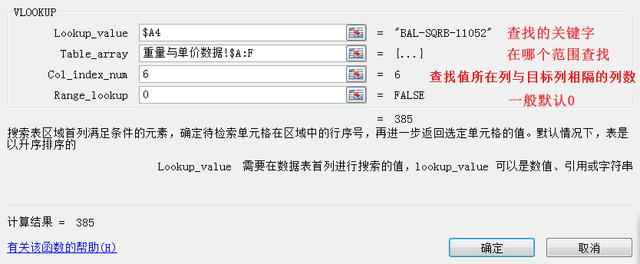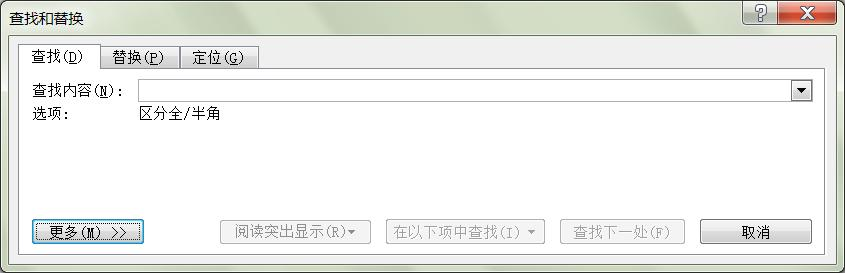excel的lookup与word的查找不同的是，word是查找到值本身所在的位置进行操作，excel是查找到value所在的位置，同时也是地址（行+列）后，是要去匹配相同行某一列的值（vlookup)或相同列某一行的值（hlookup)。所以Excel的的lookup区分vlookup和hlookup，分别去指定是第几列或第几行。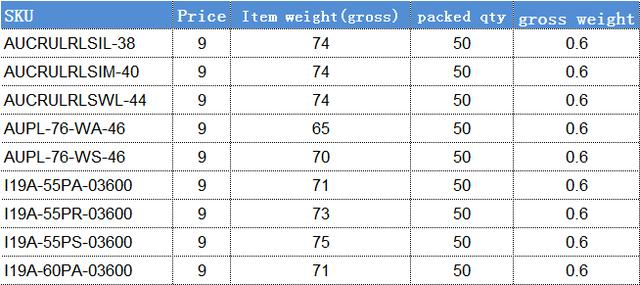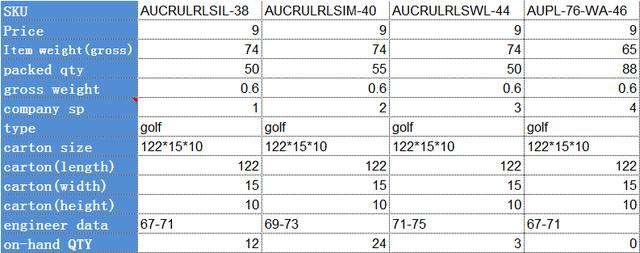vlookup函数的语法规则如下：

VLOOKUP(lookup_value,table_array,col_index_num,range_lookup)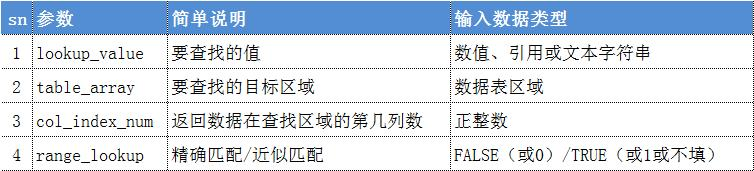1 vlookup是index+match函数的语法糖

index()可以通过行号和列号返回某一个单元格的值。

match()函数可以返回某一个值在某一行中的列号或某一列中的行号。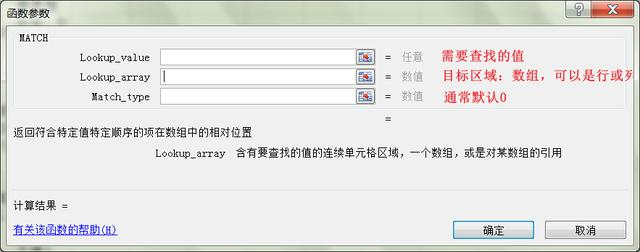=VLOOKUP(B:B,基础数据!A:D,4,0)

=INDEX(基础数据!A:D,(MATCH(B3,基础数据!A:A,0)),4)

2 vlookup函数无需修改复制到其它列

=VLOOKUP(\$A4,baseData!\$A:\$F,MATCH(C\$2,baseData!\$1:\$1,0)-0,0)

3 对于查找值，也可以使用函数来操作，如mid函数

Excel｜函数vlookup可用index+match同等实现

Vlookup函数一次性查找多个值，结合这个Match函数太方便了

vlookup函数和mid嵌套使用？

Excel｜Vlookup用常量数组实现需要查找的数据源

Excel｜深入理解数组公式及理解vlookup逆向查找的原理

Excel｜IF函数构建二维内存数组以及vlookup多值、反向、模糊查找

－End－